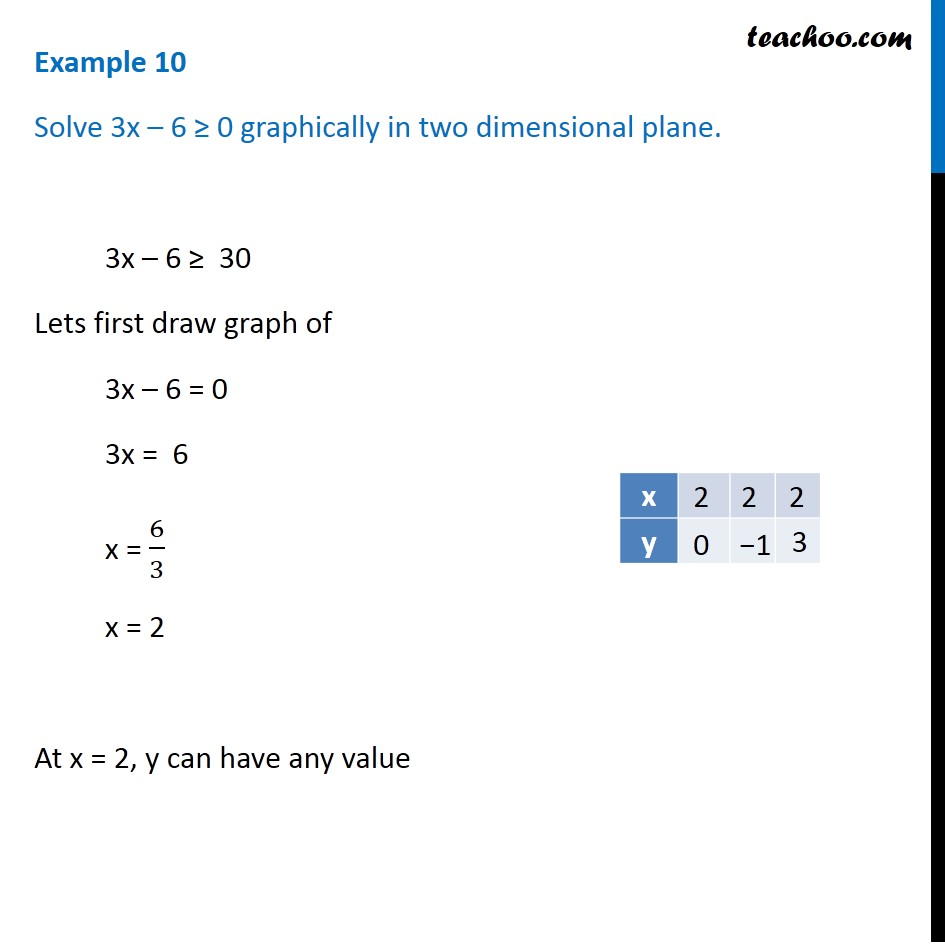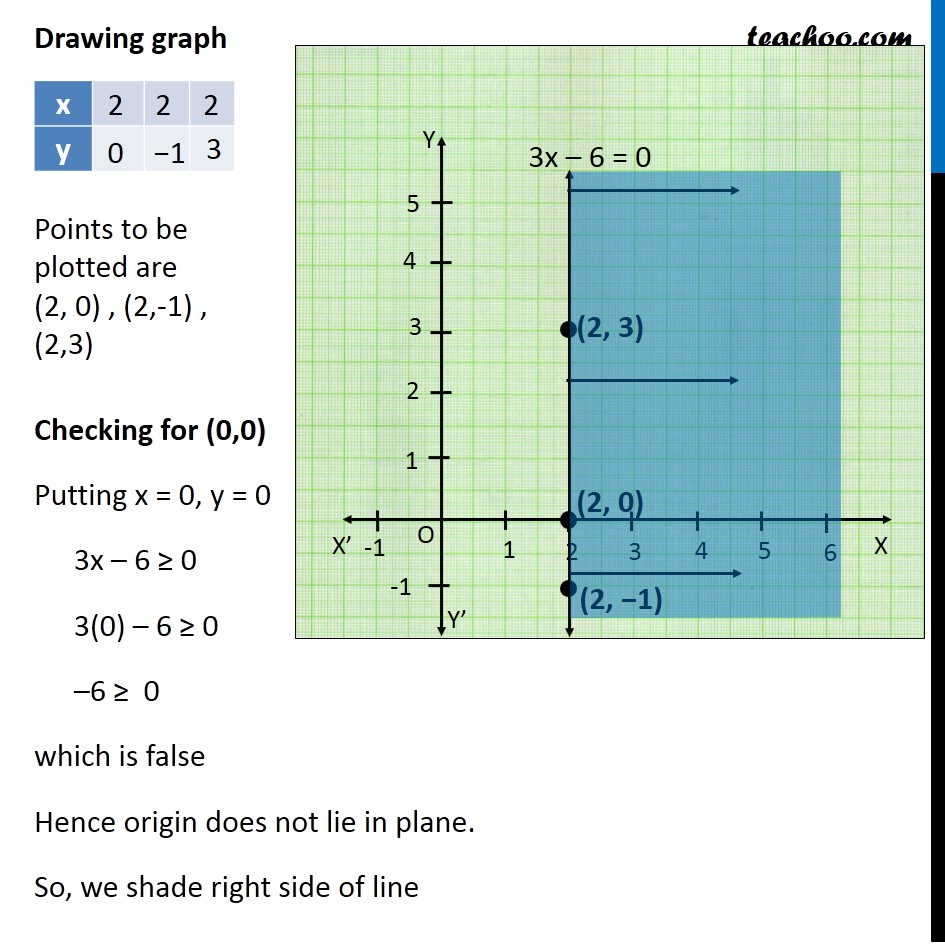Examples

Chapter 5 Class 11 Linear Inequalities
Serial order wiseLearn in your speed, with individual attention - Teachoo Maths 1-on-1 Class

### Transcript

Question 2 Solve 3x – 6 ≥ 0 graphically in two dimensional plane. 3x – 6 ≥ 30 Lets first draw graph of 3x – 6 = 0 3x = 6 x = 6/3 x = 2 At x = 2, y can have any value Points to be plotted are (2, 0) , (2,-1) , (2,3) Drawing graph Checking for (0,0) Putting x = 0, y = 0 3x – 6 ≥ 0 3(0) – 6 ≥ 0 –6 ≥ 0a which is false Hence origin does not lie in plane. So, we shade right side of line